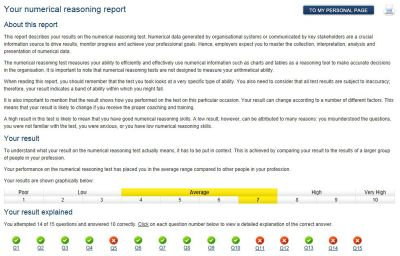Flags

# What to expect in the numerical aptitude test or numerical reasoning test

In the numerical reasoning test, you are given tables and graphs describing different business-related situations. Each business situation is followed by a number of questions (between two and five) relating to the information presented. Each question has between four and five answers. Your task is to decide for each question which is the correct answer based solely on the numerical information presented.

The numerical reasoning test is timed and, although different tests may have different time limits, you should expect to be given approximately 50– 60 seconds per question. The numerical reasoning test is designed so only 1% to 2% of test-takers can correctly answer all questions within the time limit, with the most difficult questions placed at the end of the test.

The numerical reasoning test doesn’t measure your arithmetical skills hence, you are usually allowed to use a simple calculator. The information whether you are allowed to use a calculator or not will always be given to you prior to taking the test.  In most cases, you will not even need to use a calculator to solve the test questions.

See below some numerical aptitude practice test questions examples. You can click on the image to enlarge.

•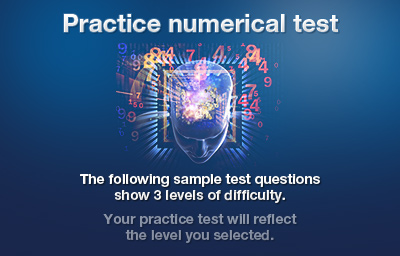•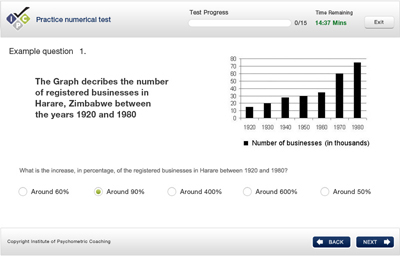•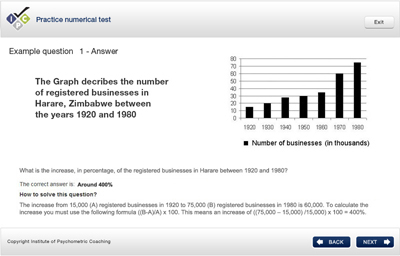•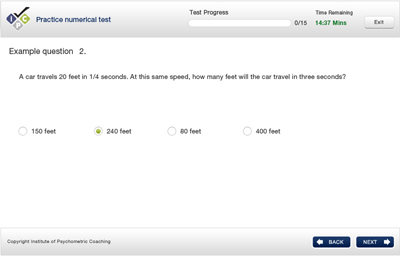•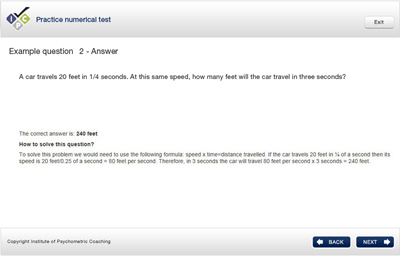•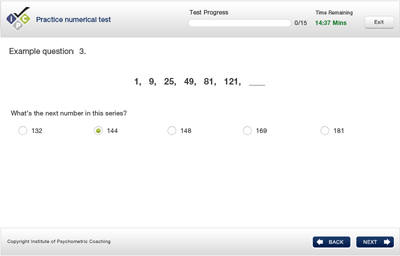•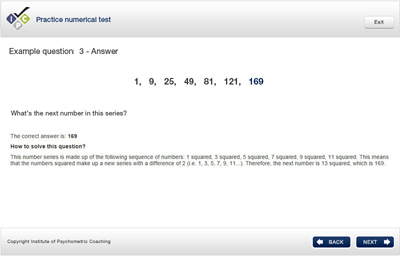•